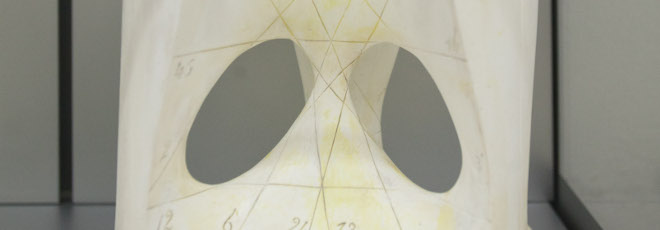## “Lower Bounds for Maximal Matchings and Maximal Independent Sets”

Giovedì 9 Gennaio 2020, ore 15:00 - Aula 2BC60 - Dennis Olivetti (University of Freiburg)

Abstract

There are distributed graph algorithms for finding maximal matchings and maximal independent sets in $O(\Delta + \log^* n)$ communication rounds; here $n$ is the number of nodes and $\Delta$ is the maximum degree. The lower bound by Linial (1987, 1992) shows that the dependency on $n$ is optimal: these problems cannot be solved in $o(\log^* n)$ rounds even if $\Delta = 2$.

However, the dependency on $\Delta$ is a long-standing open question, and there is currently an exponential gap between the upper and lower bounds.

We prove that the upper bounds are tight. We show that maximal matchings and maximal independent sets cannot be found in $o(\Delta + \log \log n / \log \log \log n)$ rounds with any randomized algorithm in the LOCAL model of distributed computing.

As a corollary, it follows that there is no deterministic algorithm for maximal matchings or maximal independent sets that runs in $o(\Delta + \log n / \log \log n)$ rounds; this is an improvement over prior lower bounds also as a function of~$n$.

Short bio

Dennis Olivetti received his Ph.D. in Computer Science in 2017 at Gran Sasso Science Institute, Italy, under the guidance of Prof. Pierre Fraigniaud.

From January 2018 to December 2019 he was a postdoc at Aalto University, Finland, in the group of Prof. Jukka Suomela. He will soon start as a postdoctoral researcher at the University of Freiburg, Germany, in the group of Prof. Fabian Kuhn. He is interested in several aspects of the theory of distributed computing, in particular in the study of the LOCAL and CONGEST models.